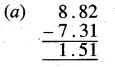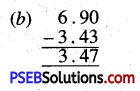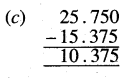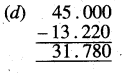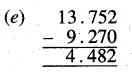# PSEB 5th Class Maths Solutions Chapter 4 Fractions Ex 4.8

Punjab State Board PSEB 5th Class Maths Book Solutions Chapter 4 Fractions Ex 4.8 Textbook Exercise Questions and Answers.

## PSEB Solutions for Class 5 Maths Chapter 4 Fractions Ex 4.8

Question 1.
(a) 2.4, 5.3 and 4.1
(b) 6.25, 5.65 and 3.01
(c) 4.32, 2.320 and 7.038
(d) 8.4, 7.03 and 2.432
(e) 12, 13.8 and 8.120.
Solution: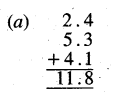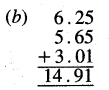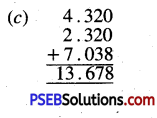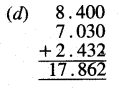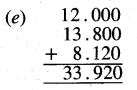Question 2.
Find the difference of the following decimals numbers :
(a) 8.82 and 7.31
(b) 6.9 and 3.43
(c) 25.750 and 15.375
(d) 45 and 13.220.
(e) 13.752 and 9.27.
Solution: